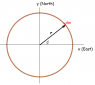# Coriolis Torque On a Spinning Object

• Dextrine

## Homework Statement

The Coriolis force can produce a torque on a spinning object. To illustrate this, consider a horizontal hoop of mass m and radius r spinning with angular velocity w about its veritcal axis at colatitude theta. Show that the Coriolis force due to the Earth's rotation produces a torque of magnitude mwWr^2sin[theta] directed to the west, where W is the Earth's angular velocity.

## Homework Equations

Coriolis force = 2mr' X W
Torque = F X d

## The Attempt at a Solution

r'=rXw

Fcor= 2m(rXw) X W
= 2mrwW

Torque = Fcor X r
= 2mr^2wWsin[theta]

I'm supposed to get half of this value somehow, and the direction is not at all clear and from my diagram is constantly changing...

Last edited by a moderator:

## Homework Equations

Coriolis force = 2mr' X W[/B]
This equation is for a particle of mass m moving with linear velocity ##\dot{\mathbf{r}}##. Each mass element of the hoop should be treated as a particle with its own velocity vector. (Different mass elements have different position and velocity vectors.)

This equation is for a particle of mass m moving with linear velocity ##\dot{\mathbf{r}}##. Each mass element of the hoop should be treated as a particle with its own velocity vector. (Different mass elements have different position and velocity vectors.)

I thought that rewriting r' as w X r with both w and r being vectors, would take care of this? If not, how would I go about treating each point independently?

You'll need to introduce a coordinate system and express the position and velocity vectors of a mass element with respect to the coordinate system. For example, you could introduce Cartesian axes with origin at the center of the hoop and with the z axis along the axis of rotation of the hoop, the x-axis pointing east, and the y-axis pointing north.

A mass element of the hoop will be at some angle ##\phi## to the x-axis. How would you express the position and velocity vectors of this mass element?

#### Attachments

•hoop on earth.png
2.9 KB · Views: 1,000
Last edited:
You'll need to introduce a coordinate system and express the position and velocity vectors of a mass element with respect to the coordinate system. For example, you could introduce Cartesian axes with origin at the center of the hoop and with the z axis along the axis of rotation of the hoop, the x-axis pointing east, and the y-axis pointing north.

A mass element of the hoop will be at some angle ##\phi## to the x-axis. How would you express the position and velocity vectors of this mass element?

[r cos[phi] x direction + r sin[phi] y direction] X w z direction

[r cos[phi] x direction + r sin[phi] y direction] X w z direction
I'm guessing that this is an expression for the velocity vector of the mass element located at angle ##\phi##. Does the expression have the correct overall sign? Can you simplify by carrying out the cross product?

Note that math symbols are available by clicking on the ##\Sigma## tab.

Thank you for that, I couldn't remember how i had made symbols before. Anyway, I understand it now, thanks a lot!